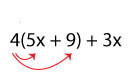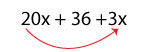# How to Simplify Algebraic Expressions

1. Math Lessons >
2. Simplifying Algebraic Expressions

What is simplifying?

To put it simply, the word “simplify” means to make something simple and easy to understand. Since algebraic expressions are largely dreaded by students, the idea is we should teach the topic as simply as it could be, and thankfully “Simplifying Algebraic Expressions” helps the cause by making calculations easier like never before.

Here's how you simplify an algebraic expression; first remove symbols of grouping (parentheses or brackets) and then combine the like terms. The process of simplification is instrumental in changing a complex expression into a simple expression.

How do you simplify an algebraic expression?

To break down an expression into the simplest form, you can:

1. Combine the like terms

Like terms – Like terms have the same variables and exponents.

5x + 2x

= 7x

Unlike terms – Unlike terms do not have the same variables and powers. Unlike terms cannot be combined.

5x + 3x2

As there are no like terms in the expression, you cannot combine them.

2. Use Distributive Property

You can simplify an expression by removing parentheses. The distributive property allows you to multiply a number outside the parentheses to the number inside the parentheses, resulting in a simplified version of the expression.

Simplify 4(5x + 9) + 3xApplying the distributive property, we getCombining the like terms, we obtain

23x + 36

The lesson in a nutshell:

Apply the distributive property to remove parentheses.

Add the coefficients of the same variables and exponents to combine the like terms.

Terms without coefficient or terms containing only variables have a coefficient of 1.

Simplify fractions if required.Put your skills into prolific practice, using our free printable Simplifying Algebraic Expression worksheets.

Solved Examples:

Example 1

1. Simplify 3c + 15e – 4e + 5c + 8c

3c + 15e – 4e + 5c + 8c

= 3c + 5c + 8c + 15e – 4e

[ Grouping like terms together ]

= 16c + 11e

[ Combining like terms ]

16c + 11e is the simplest form of the expression 3c + 15e – 4e + 5c + 8c.

Example 2

2. Simplify n + 5(n + 5) + 7n

n + 5(n + 5) + 7n

= n + 5n + 25 + 7n

[ Applying distributive property ]

= n + 5n + 7n + 25

[ Grouping like terms ]

= 13n + 25

[ Combining like terms ]

13n + 25 is the simplest form of the expression n + 5(n + 5) + 7n.

Example 3

3. Simplify 2(x + 5) – 3(y + 6)

2(x + 5) – 3(y + 6)

= 2x + 10 – 3y – 18

[ Applying distributive property ]

= 2x – 3y – 8

[ Combining like terms ]

2x – 3y – 8 is the simplest form of the expression 2(x + 5) – 3(y + 6).